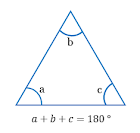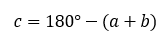## C program to find angles of triangle if two angles are given

Write a C Program to input two angles from user and find third angle of the triangle. How to find all angles of a triangle if two angles are given by user using C programming. C program to calculate the third angle of a triangle if two angles are given.

#### Required knowledge

C Operators, Data Types in c, Basic input/output

#### Properties of triangle

Sum of angles of a triangle is 180°.Let us apply basic math to derive formula for third angle. If two angles of a triangle are given, then third angle of triangle is given by -

formula for third angle of a triangle#### Logic to find third angle of a triangle

Step by step descriptive logic to find third angle of a triangle -

1.Input two angles of triangle from user. Store it in some variable say a and b.

2.Compute third angle of triangle using formula c = 180 - (a + b).

3.Print value of third angle i.e. print c.

#### Program to find angles of a triangle

```
/**
* C program to find all angles of a triangle if two angles are given
*/

#include <stdio.h>

int main()
{
int a, b, c;

/* Input two angles of the triangle */
printf("Enter two angles of triangle: ");
scanf("%d%d", &a, &b);

/* Compute third angle */
c = 180 - (a + b);

/* Print value of the third angle */
printf("Third angle of the triangle = %d", c);

return 0;
}
```

##### Output:
```
Enter two angles of triangle: 60 30
Third angle of the triangle = 90
```
```
Recommended posts

Loop programming exercises index.
Basic programming exercises index.
If else programming exercises index.
Array programming exercises index.
String programming exercises index.
Pointer programming exercises index.
Star Patterns exercises index.
Number Patterns exercises index.
alphabet Patterns exercises index.
series program exercises index.

```